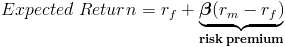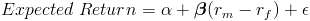# Capital Asset Pricing Model (CAPM)

CAPM Calculator (Click Here or Scroll Down)The capital asset pricing model provides a formula that calculates the expected return on a security based on its level of risk. The formula for the capital asset pricing model is the risk free rate plus beta times the difference of the return on the market and the risk free rate.

## Risk and the Capital Asset Pricing Model Formula

To understand the capital asset pricing model, there must be an understanding of the risk on an investment. Individual securities carry a risk of depreciation which is a loss of investment to the investor. Some securities have more risk than others and with additional risk, an investor expects to realize a higher return on their investment. For example, assume that an individual has \$100 and two acquaintances would like to borrow the \$100 and both are offering a 5% return(\$105) after 1 year. The obvious choice would be to lend to the individual who is more likely to pay, i.e., carries less risk of default. The same concept can be applied to the risk involved with securities.

The risk involved when evaluating a particular stock is accounted for in the capital asset pricing model formula with beta. Specifically regarding the capital asset pricing model formula, beta is the measure of risk involved with investing in a particular stock relative to the risk of the market. The beta of the market would be 1. An individual security with a beta of 1.5 would be as proportionally riskier than the market and inversely, a beta of .5 would have less risk than the market.

## Risk Free Rate in the Capital Asset Pricing Model Formula

The risk free rate would be the rate that is expected on an investment that is assumed to have no risk involved. For the US, the US treasury bill rate is generally used as it is short term and the collapse of the treasury bill would theoretically, at minimum, be a large enough disruption to inhibit gauging value, or at worse, be a collapse of the entire monetary system which relies on a fiat currency.

## Risk Premium in the Capital Asset Pricing Model Formula

The capital asset pricing model formula can be broken up into two components: the risk free rate and the risk premium of the particular security.The risk premium is beta times the difference between the market return and a risk free return. In the capital asset pricing model formula, by subtracting the market return from a risk free return, the risk of the overall market can then be determined. By multiplying beta times this risk of the market, the risk of the individual stock can then be determined. As previously stated, beta is the risk of an individual security relative to the market. A beta of 2 would be twice as risky as the market. In practice, risk is synonymous with volatility. A stock with a beta larger than the market beta of 1 will generally see a greater increase than the market when the market is up and see a greater decrease than the market when the market is down.

## Alternative Capital Asset Pricing Model FormulaWhen regression analysis is applied to the capital asset pricing model based on prior returns, the formula will be shown as above. Alpha is considered to be the risk free rate and epsilon is considered to be the error in the regression.

New to Finance?